# Introduction

Modern biological experiments are increasingly producing interesting matrices. These may represent the presence or absence of specific gene mutations, copy number variants, microRNAs, or other molecular or clinical phenomena. We have recently developed a tool, CytoGPS [^Abrams and colleagues], that converts conventional karyotypes from the standard text-based notation (the International Standard for Human Cytogenetic Nomenclature; ISCN) into a binary vector with three bits (loss, gain, or fusion) per cytoband, which we call the “LGF model”.

The Mercator package is intended to facilitate the exploration of data sets. It implements metrics for continuous, categorical, and binary data, including a subset of the 76 binary distance metrics described by [^Choi and colleagues], ensuring that at least one representative of each of their major clusters is included. Each resulting distance matrix can be combined with multiple visualization techniques, providing a consistent interface to thoroughly explore the data set.

# The BinaryMatrix Class

In this vignette, we focus initially on the tools for dealing with binary matrices. We start by loading the package.

suppressMessages( suppressWarnings( library(Mercator) ) )

## A Limited Sample Dataset

We proceed with a model dataset of karyotypes from patients with Chronic Myelogenous Leukemia (CML) with 400 chromosomal features recorded over 740 patients, from the public Mitelman database. Cytogenetic abnormalities recorded in Mitelman as text strings have been pre-processed with CytoGPS into binary vectors. For the sake of clarity and efficiency we have chosen a subset of patients and features for this example.

filename <- system.file("Examples/Mercator_Test_Data.csv", package="Mercator")
my.data <- read.csv(filename, header=TRUE)
dim(my.data)
##  740 400

## Generating the BinaryMatrix

Many of the functions of the Mercator package operate on a BinaryMatrix S4 object, which serves as an input to subsequent functions and visualizations.

A BinaryMatrix object is formed from a matrix containing integer or numeric values. Although Mercator was intially designed for processing and visualizing binary data, the BinaryMatrix object and subsequent functions accept a variety of integer and numeric values. In addition, all the visualization tools work with an arbitrary distance matrix; see the vignette Mercator for Continuous Data.

Row and column names, along with optional annotations, can be assigned as for data frames. If no row or column headings are assigned, the BinaryMatrix takes the row and column names of the input matrix by default. Notice that the resulting object always includes a “history” element that tracks how it has been processed.

my.data <- as.matrix(my.data)
my.binmat <- BinaryMatrix(my.data)
summary(my.binmat)
## An object of the 'BinaryMatrix' class, of size
##  740 400
## History:
##  "Newly created."

We wish to cluster the whole karyotypes of each patient to identify the patterns of important chromosomal abnormalities that link them. To proceed, we must transpose the BinaryMatrix. Transposition meaningfully transposes the row and column headings of the BinaryMatrix, as well.

my.binmat <- t(my.binmat)
summary(my.binmat)
## An object of the 'BinaryMatrix' class, of size
##  400 740
## History:
##  "Newly created." "transposed"

## Remove Duplicates

The binary vectors are rarely unique. Patients who have identical vectors of features can complicate some of the clustering and visualization routines that we want to use (often by introducing a division by zero). Similarly, features that have identical occurence vectors in a patient cohort can also alter the biological implications by automatically giving more “weight” to a single genomic event (like a trisomy or monosomy). To deal with this issue, the Mercator package includes a function to remove duplicate or redundant binary vectors. Here we are going to remove duplicate patients; that is, those with identical karyotypes.

my.binmat <- removeDuplicates(my.binmat)
summary(my.binmat)
## An object of the 'BinaryMatrix' class, of size
##  400 136
## History:
##  "Newly created."      "transposed"          "Duplicates removed."

In the case of our data, only 136 of the 740 patient karyotypes are unique. Some of the karyotypes are “not used” in the sense that they contain none of the abnormalities selected for this limited subset.

length(my.binmat@info$notUsed) ##  65 head(my.binmat@info$notUsed)
##  "R17" "R18" "R23" "R34" "R44" "R51"

By contrast, many features are used but are simply redundant.

length(my.binmat@info\$redundant)
##  539

## Data Filtering with Thresher

Mercator provides easy access to functions of the Thresher R package, which includes outlier detection and estimates of the number of clusters [^Wang and colleagues]. The underlying idea is that the features can be viewed as “weight vectors” in a principal component space trying to display the samples. The lengths of the vectors are a measure of their importance in the data set; short vectors can (and probably should) be removed since they do not carry much useful information. We have incorporated that feature into the Mercator package.

We create a ThreshedBinaryMatrix to implement the algorithm. Based on simulations described in the Thresher paper, a cutoff above approximately 0.3 can be used to select informative features. Then, we subset our ThreshedBinaryMatrix to only include features above our given cutoff.

set.seed(21348)
my.binmat <- threshLGF(my.binmat, cutoff=0.3)
summary(my.binmat)
## An object of the 'BinaryMatrix' class, of size
##  400 111
## History:
##  "Newly created."      "transposed"          "Duplicates removed."
##  "Threshed."

The red vertical line in the figure indicates the cutoff we have chosen to separate uninformative features (<0.3) from informative ones (>0.3).

Delta <- my.binmat@thresher@delta
hist(Delta, breaks=20, main="", xlab="Weight", col="gray")
abline(v=0.3, col='red')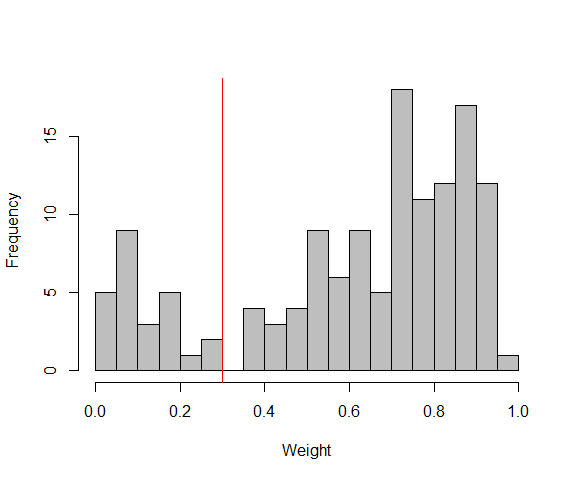Histogram of weight vectors.

The ThreshedBinaryMatrix object contains a reaper slot that estimates the number of principal components and the number of clusters after outliers have been removed. These values can be viewed numerically…

my.binmat@reaper@pcdim
##  2
my.binmat@reaper@nGroups
##  5

… or they can be visualized with an Auer-Gervini plot (where we are looking for a “long step”) …

plot(my.binmat@reaper@ag, ylim=c(0, 30))
abline(h=my.binmat@reaper@pcdim, col="forestgreen", lwd=2)
abline(h=7, col="orange", lwd=2)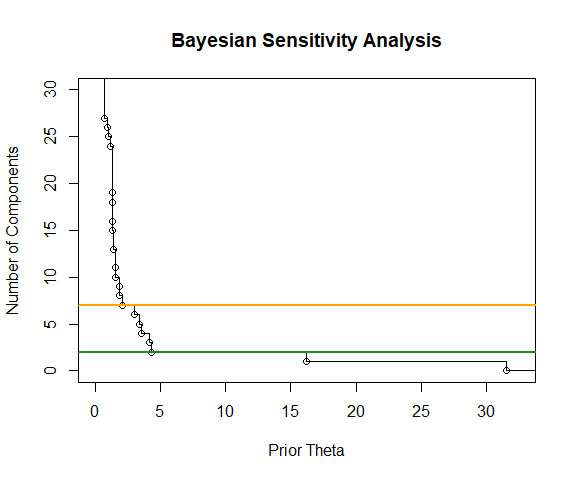Auer-Gervini plot.

pts <- screeplot(my.binmat@reaper, xlim=c(0,30))
abline(v=pts[my.binmat@reaper@pcdim], col="forestgreen", lwd=2)
abline(v=pts, col="orange", lwd=2)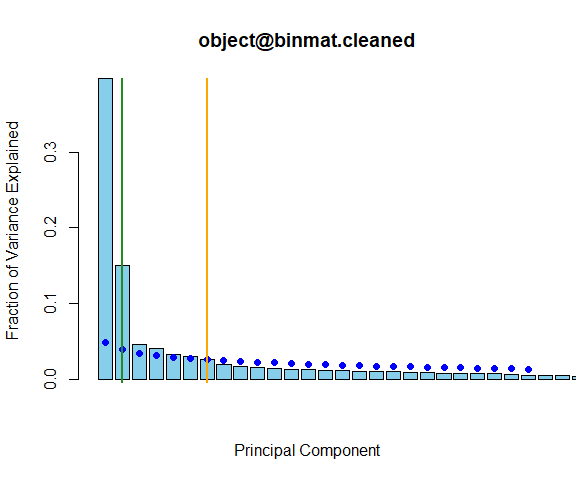Scree plot.

There is no method that can definitely identify the number of clusters for a “perfect” solution, leaving part of the decision to the analyst’s judgment. Auer-Gervini and Scree plots provide informative visualizations to facilitate this interference. The default value provided by the Auer-Gervini analysis (N=2; green) is somewhat conservative. The value provided by the broken-stick model (N=7; orange) overlaid on the scree plot is more aggressive, but is not too unreasonable based on the Auer-Gervini plot. While one could make the argument that the correct number of groups is at least seven, we will begin by using the number of groups recommended by Thresher.

kk <- 5

# Visualization

The Mercator package allows visualization of data with methods that include both standard techniques (hierarchical clustering) and large-scale visualizations (multidimensional scaling (MDS), t-distributed Stochastic Neighbor Embedding (t-SNE), and iGraph.)

The Mercator package implements or provides access to 10 distance metrics: Jaccard, Sokal-Michener, Hamming, Russell-Rao, Pearson, Goodman-Kruskal, Manhattan, Canberra, Binary, and Euclidean. Although some of these metrics can be used for continuous or categorical data, all are appropriate for binary matrices. Mercator allows the user to compare different metrics and select one that is useful for a given dataset.

## Jaccard Distance

Here, we will use the Jaccard distance because of its ease of interpretability, common usage, and its appropriatness of application to asymmetric binary data, such as the binary vector output of CytoGPS in this dataset. The Mercator constructor can be called with any initial visualization, and visualizations can be added in an arbitrary order.

jacc.Vis <- Mercator(my.binmat, "jaccard", "hclust", K=kk)

We can represent all the distances between features within the dissimilarity matrix we have calculated on our data as a histogram, as a visual representation of relatedness.

hist(jacc.Vis,
xlab="Jaccard Distance", main="Histogram of Distances")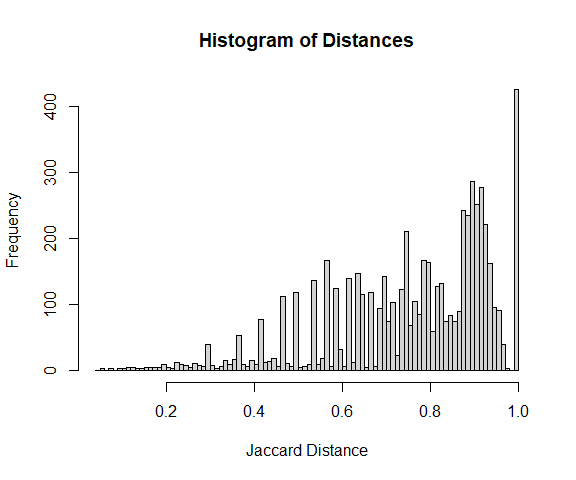Distribution of Jaccard distances.

### Hierarchical Clustering

Mercator allows us to implement common, standard visualizations such as hierarchical clustering…

plot(jacc.Vis, view = "hclust")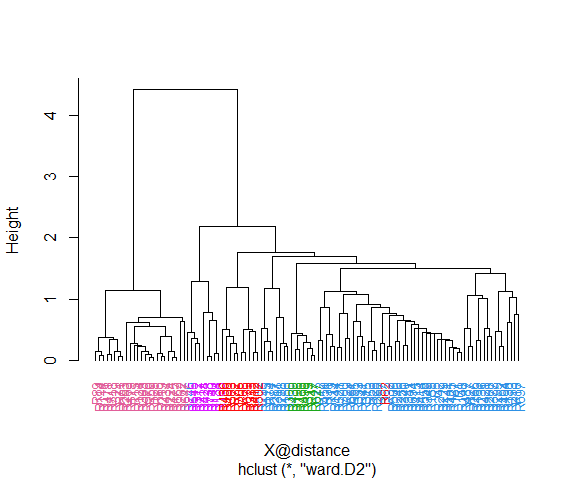Hierarchical clustering using Jaccard distances.

In this dendrogram, there appear to be at least three different “blue” branches, suggesting that there are more than the conservative estimate of five clusters obtained from Thresher. We will take a look at the data using another visualization technique before making a final decision.

### t-Distributed Stochastic Neighbor Embedding

Mercator can use t-distributed Stochastic Neighbor Embedding (t-SNE) plots for visualizing large-scale, high-dimensional data in 2-dimensional space.

jacc.Vis <- addVisualization(jacc.Vis, "tsne", perplexity=25)
plot(jacc.Vis, view = "tsne", main="t-SNE; Jaccard Distance")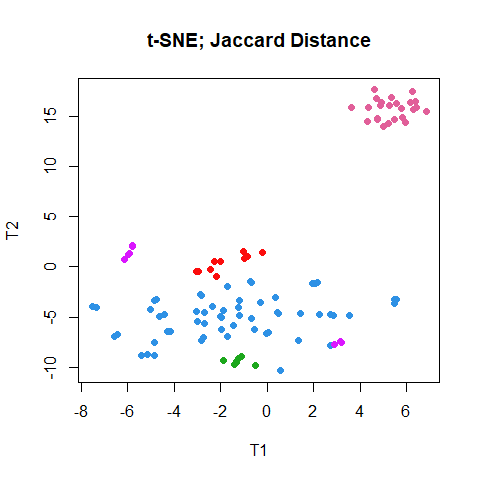A t-SNE plot.

Optional t-SNE parameters, such as perplexity, can be used to fine-tune the plot as the visualization is created. Using addVisualization to create a new, tuned plot of an existing type overwrites the existing plot of that type.

temp.Vis <- addVisualization(jacc.Vis, "tsne", perplexity = 10)
## Warning in addVisualization(jacc.Vis, "tsne", perplexity = 10): Overwriting an
## existing visualization:tsne
plot(temp.Vis, view = "tsne",  main="t-SNE; Jaccard Distance; perplexity=10")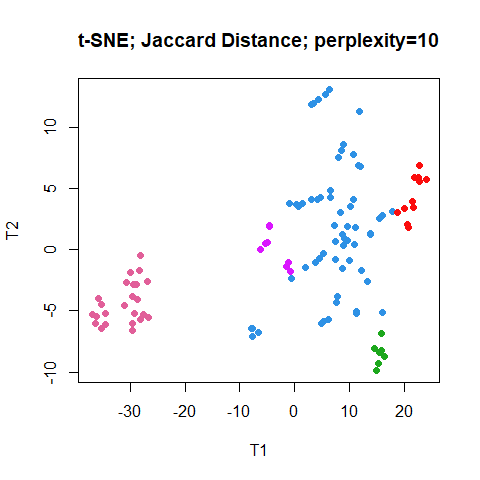Another t-SNE plot.

It does not appear that a smaller perplexity is useful for this data set.

### Multi-Dimensional Scaling

Mercator allows visualization of multi-dimensional scaling (MDS) plots, as well.

jacc.Vis <- addVisualization(jacc.Vis, "mds")
plot(jacc.Vis, view = "mds", main="MDS; Jaccard Distance")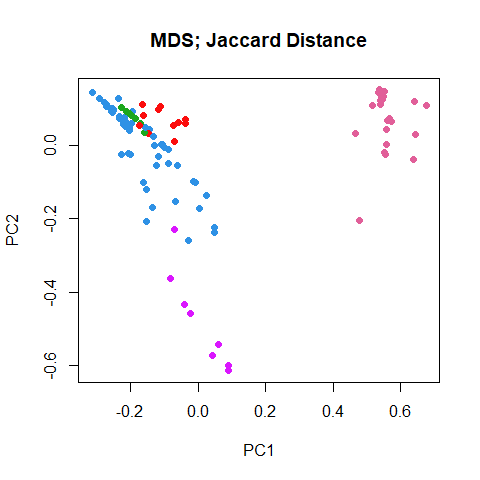A multi-dimensioanl scaling plot.

### Silhouette-Width Barplots

At this point, we still don’t know if we should increase the number of clusters in the data set form 5 to 7. Fortunately, we have access to the “silhouette width”, which measures how well each element in the data set appears to be clustered.

barplot(jacc.Vis)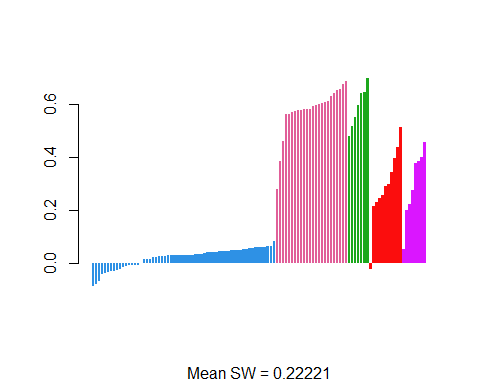Histogram of silhouette widths.

Ths plot of the silhouette widths confirms our suspicion from the hierarchical clustering dendrogram that the “blue” group is not as coherent/consistent as the other grousp. So, we are going to try reclustering to label more clusters.

jacc.Vis6 <- recluster(jacc.Vis, K = 6)
barplot(jacc.Vis6)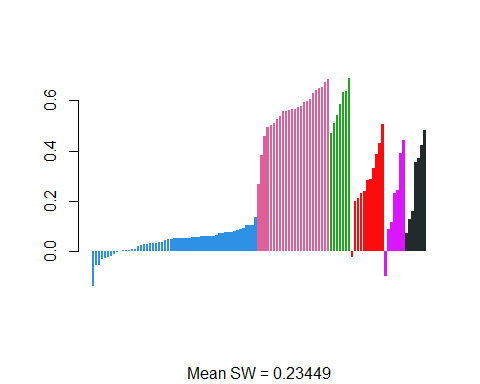Silhouette widths for different K.

jacc.Vis7 <- recluster(jacc.Vis, K = 7)
barplot(jacc.Vis7)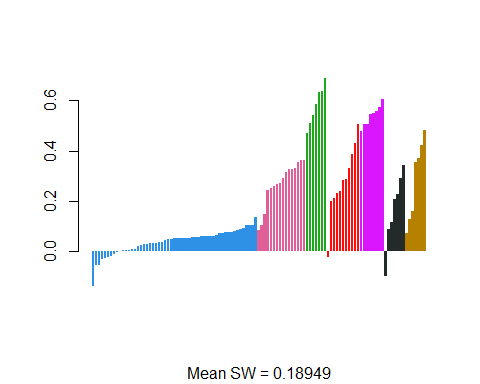Silhouette widths for different K.

There is some improvement when K=6, but a clearly worse result when K = 7. For the rest of our analysis, we will switch to ooking at 6 groups.

kk <- 6
jacc.Vis <- jacc.Vis6
rm(jacc.Vis6, jacc.Vis7)

### iGraph

Mercator can be used to visualize complex networks using iGraph. To improve clarity of the visualization and computational time, we have implement the downsample function to reduce the number of data points to be linked and visualized. The idea goes back to Peng Qiu’s implementation of the SPADE clustering algorithm for mass cytometry data. The main point is to under sample the densest regions of the data space to make it more likely that rarer clusters will still be adequately sampled. (While not required with the current data set, this idea can be quite useful with data sets containing tens of thousands of objects.)

Note: The Mercator class includes a “subset” operator that tries to preserve earlier visualizations. This operator is fast for MDS or t-SNE models, but is very slow for large hierarchical clustering. (It uses the implementation in the dendextend package, which works by removing a single leaf at a time from the tree.) In the next code chunk, we downsample to create a meaningful subset, and then compute a new dendrogram for each subset.

X <- jacc.Vis
X@view[["hclust"]] <- NULL # remove this view
N <- as.matrix(X@distance)
set.seed(87530)
P <- downsample(40, N, 0.1) # create a downsampled subset
J <- X[P]
names(J@view) # need to compute a new dendrogram
##  "tsne" "mds"
J <- addVisualization(J, "hclust", perplexity=5)
names(J@view)
##  "tsne"   "mds"    "hclust"
plot(J, view = "tsne", main="Down-sampled t-SNE Plot")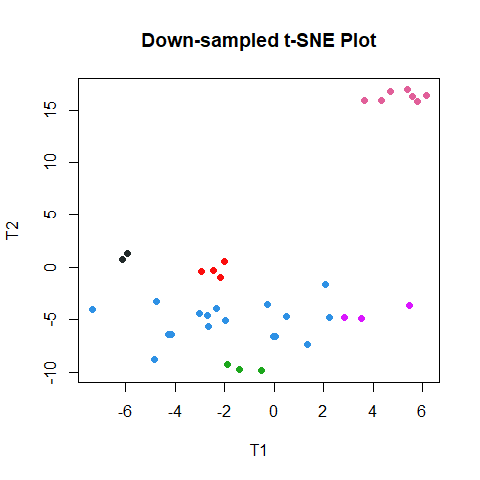Downsample t-SNE plot.

Because our data set is small enough, we are going to create our graphical views from the oriinal object rather than the downsampled one. We can look at the resulting graph using three different “layouts”.

jacc.Vis <- addVisualization(jacc.Vis, "graph", Q =0.5)
plot(jacc.Vis, view = "graph", layout = "tsne", main="T-SNE Layout")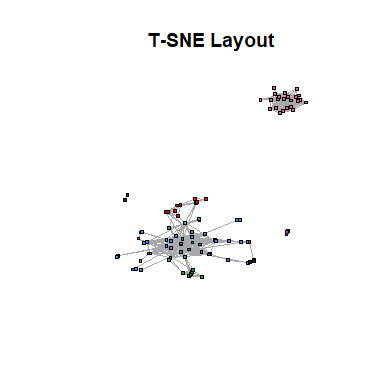Networks.

plot(jacc.Vis, view = "graph", layout = "mds", main="MDS Layout")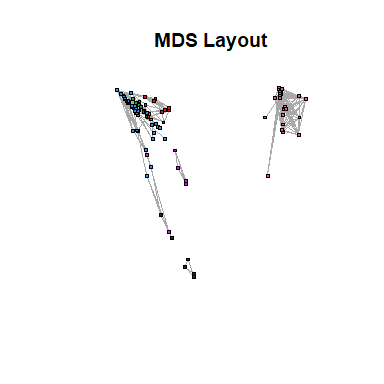Networks.

plot(jacc.Vis, view = "graph", layout = "nicely",
main="Laid Out 'Nicely'",
xlim=c(-1,1))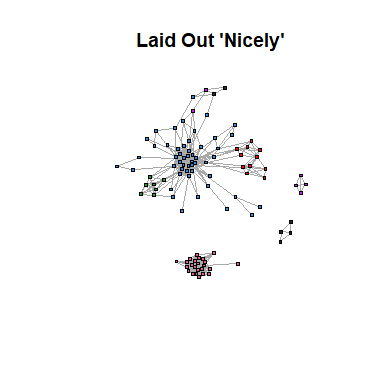Networks.

## Cluster Identities

We can use the getClusters function to characterize each cluster and use these for further manipulation.

We can easily determine cluster size…

my.clust <- getClusters(jacc.Vis)
tab <- table(my.clust)
tab
## my.clust
##  1  2  3  4  5  6
## 55 24  7 11  7  7

… or the patients that comprise each cluster.

C <- my.binmat@columnInfo
Cl4 <- C[my.clust == 4 ,]
Cl4
##   "R62"  "R68"  "R72"  "R108" "R333" "R412" "R436" "R502" "R503" "R679"
##  "R705"

## Sokal-Michener Metric

Finally, we are going to look at what happens if we use a different distance metric.

set.seed(8642)
sokal.Vis <- Mercator(my.binmat, "sokal", "tsne", K=kk, peplexity = 10)
table(getClusters(sokal.Vis), getClusters(jacc.Vis))
##
##      1  2  3  4  5  6
##   1 55  0  7 11  3  3
##   2  0 16  0  0  0  0
##   3  0  8  0  0  0  0
##   4  0  0  0  0  4  0
##   5  0  0  0  0  0  2
##   6  0  0  0  0  0  2
plot(sokal.Vis, view = "tsne", main="t-SNE; Sokal-Michener Distance; perplexity=10")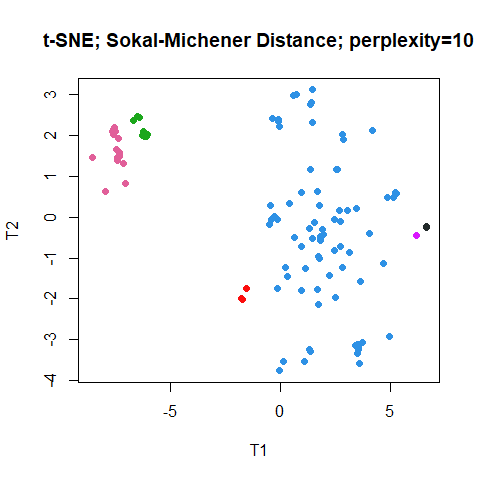Sokal-Michener distance t-SNE plot.

The two largest groups get assigned the same colors (by chance) in the Jaccard and the Sokal-Michener clusterings. However, it is not at all clear how to align the smaller groups. For that purpose we can use the remapColors function.

SV <- remapColors(jacc.Vis, sokal.Vis)
table(getClusters(SV), getClusters(jacc.Vis))
##
##      1  2  3  4  5  6
##   1 55  0  7 11  3  3
##   2  0 16  0  0  0  0
##   3  0  8  0  0  0  0
##   4  0  0  0  0  0  2
##   5  0  0  0  0  4  0
##   6  0  0  0  0  0  2
plot(SV, view = "tsne", main="t-SNE; Sokal-Michener Distance; perplexity=10")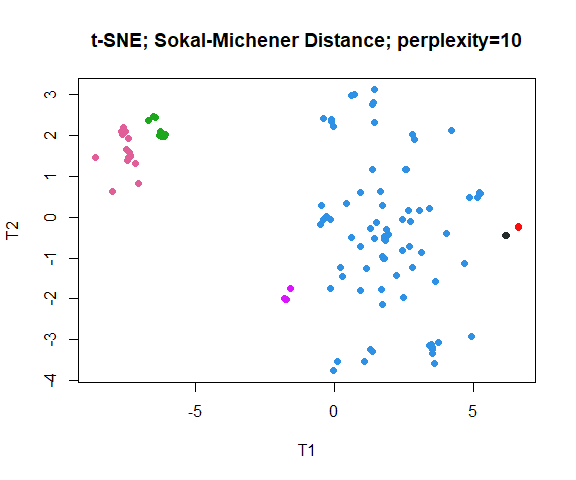Now colors have been matched (as well as possible) between the two sets of visualizations.

# Changing the Color Palette

Each Mercator object stores its own palette internally, but you can change the palette using the slot funciton.

slot(jacc.Vis, "palette") <- c("red", "orange", "green", "blue",
"cyan", "magenta", "purple", "black")
plot(jacc.Vis, view = "tsne")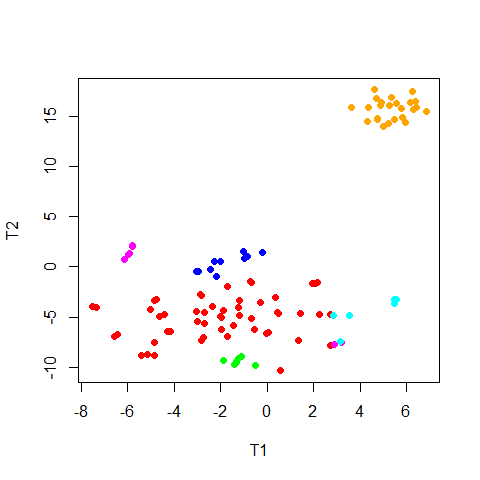Recolored Jaccard t-SNE plot.

If the number of colors in the palette is smaller than the number of clusters, they will be recycled, and other plotting symbols will be introduced.

slot(jacc.Vis, "palette") <- c("red", "green", "blue", "purple")
plot(jacc.Vis, view = "tsne")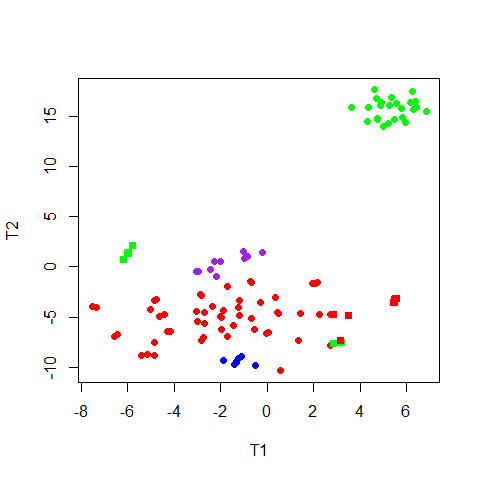Recolored Jaccard t-SNE plot.